## Print the total binary entropy

TBE (bit labels);
shows the sum of the binary entropies of individual qubits specified by bit labels. A binary entropy of the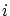-th qubit is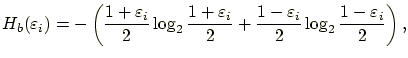(16)

where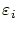is the intrinsic polarization of the-th qubit, which is calculated according to the eigenvalues,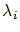and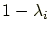, of the reduced density operator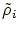of the qubit. In other words, the command shows the total binary entropy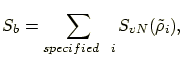(17)

where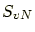denotes the von Neumann entropy.

root
2004-06-15